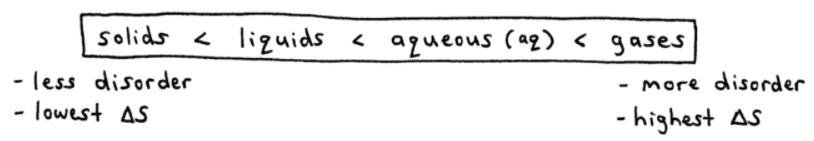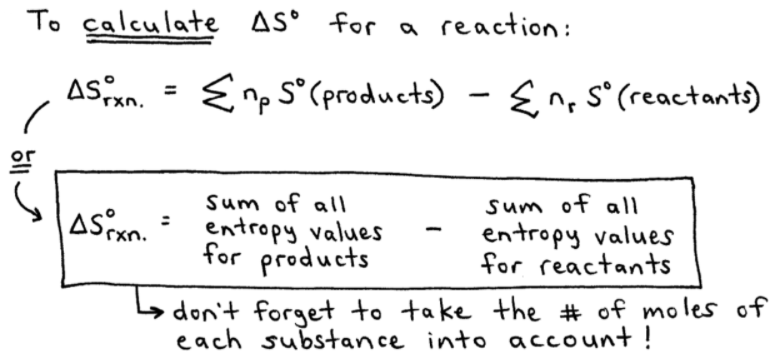# S16E2 - Entropy Changes in Chemical Reactions, and Gibbs Free Energy

## Second Law of Thermodynamics

2nd Law of Thermodynamics  =  the entropy (S) of the universe is constantly increasing. In any spontaneous process, the entropy of the universe increases.

The change in entropy of the universe is given the symbol, ΔSuniv➞ if ΔSuniv > 0 , the process is spontaneous.
➞ if ΔSuniv < 0 , the reverse process is spontaneous.
➞ if ΔSuniv = 0 , the process has no tendency to occur (it's at equilibrium).

➞ Sometimes ΔSsys and ΔSsurr have opposite signs, and their magnitudes determine whether or not the overall process is spontaneous ( ΔSsurr > 0 ).

=====

## Gibbs Free Energy, G

Gibbs Free Energy, G  =  a thermodynamic function that not only is related to spontaneity, but also deals with the temperature dependence of spontaneity:

### ΔG  =  ΔH  -  TΔS➞ if ΔG < 0 , the process is spontaneous.
➞ if ΔG > 0 , the process is not spontaneous.

➞ To calculate ΔG, you must compare and consider the ΔH and ΔS values, and you must consider the Kelvin temperature (T).

-----

ex:  At what temperatures is the following process spontaneous at 1atm?

Br2(l)   ➞   Br2(g)

ΔH° = 31.0 kJ/mol, and
ΔS° = 93.0 J/K.mol

Well, ΔG must be negative in ΔG°  =  ΔH°  -  TΔS°  (° symbol = 25°C, 1atm conditions)...

... So we can find the threshold temperature (T) by setting ΔG°  =  0NOTE - When T = 333K, the free-energy change (ΔG) = 0 and the liquid and gas phases of Br2 coexist at 1atm.

-- so, the normal boiling point (bp) = 333K

=====

## What is Entropy?

### Entropy Changes in Chemical Reactions

-- It's most important to focus on the amount of gas molecules.ex:  Does entropy increase or decrease in the following reactions?

i.     N2(g)   +   3H2(g)       2NH3(g)

- 4 gas molecules "goes to" 2 gas molecules, and fewer gas molecules means fewer possible configurations.

- fewer gas molecules  =  less disorder  =  entropy decreases, ΔS < 0ii.    4NH3(g)   +   5O2(g)       4NO(g)   +   6H2O(g)

- entropy increases, ΔS > 0, because 9 gas molecules becomes 10 gas molecules.

=====

## Standard Entropy Values

Standard Entropy Values (S°) are listed in a lengthy table or appendix in the back of your textbook. You can find one here as well: Table of Standard Entropies.

➞ notice that indeed S° increases for a substance in going from solid to a liquid to a gas.

➞ S° has units of " J / K.mol "

➞ To calculate ΔS° for a reaction:

ΔS°rxn  =  (sum of all entropies for products)  -  (sum of all entropies of reactants) , or...-----

ex:  Calculate ΔS° for this reaction:

Al2O3(s)   +   3H2(g)      2Al(s)   +   3H2O(g)

- First, we will need a Table of Standard Entropy Values (S°) from the back of our textbook, or use the link I gave you earlier in this article (above).

- This gives us...Question:  In the sample problem above, if 3 gas molecules (reactants) are converted to 3 gas molecules (products), how come the ΔS° value is large instead of nearly 0 J/K ?...

Answer:  The large ΔS° value is because H2O is more complex than H2, and thus has a higher standard entropy value (S°).

Why?  Because H2O's molecular structure, being more sophisticated, has a greater number of vibrations/rotations/etc. which increase its randomness and disorder (entropy).

=====

In my next post covering SECTION 16 - Spontaneity, Entropy, and Free Energy,

We'll talk extensively about Spontaneous Reactions and the Gibbs Free Energy Change, ΔG°